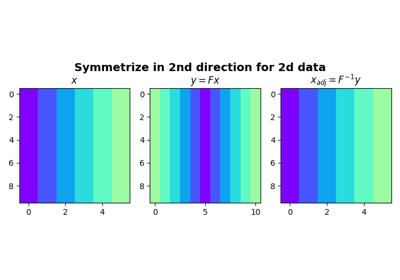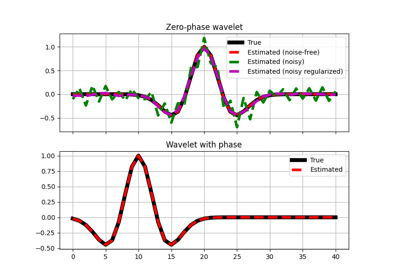# pylops.Symmetrize¶

class pylops.Symmetrize(N, dims=None, dir=0, dtype='float64')[source]

Symmetrize along an axis.

Symmetrize a multi-dimensional array along a specified direction dir.

Parameters: N : int Number of samples in model. Symmetric data has $$2N-1$$ samples dims : list, optional Number of samples for each dimension (None if only one dimension is available) dir : int, optional Direction along which symmetrization is applied dtype : str, optional Type of elements in input array

Notes

The Symmetrize operator constructs a symmetric array given an input model in forward mode, by pre-pending the input model in reversed order.

For simplicity, given a one dimensional array, the forward operation can be expressed as:

$\begin{split}y[i] = \begin{cases} x[i-N+1],& i\geq N\\ x[N-1-i],& \text{otherwise} \end{cases}\end{split}$

for $$i=0,1,2,\ldots,2N-2$$, where $$N$$ is the lenght of the input model.

In adjoint mode, the Symmetrize operator assigns the sums of the elements in position $$N-1-i$$ and $$N-1+i$$ to position $$i$$ as follows:

$\begin{multline} x[i] = y[N-1-i]+y[N-1+i] \quad \forall i=0,2,\ldots,N-1 \end{multline}$

apart from the central sample where $$x = y[N-1]$$.

Attributes: shape : tuple Operator shape explicit : bool Operator contains a matrix that can be solved explicitly (True) or not (False)

Methods

 __init__(N[, dims, dir, dtype]) Initialize this LinearOperator. adjoint() Hermitian adjoint. apply_columns(cols) Apply subset of columns of operator cond([uselobpcg]) Condition number of linear operator. conj() Complex conjugate operator div(y[, niter, densesolver]) Solve the linear problem $$\mathbf{y}=\mathbf{A}\mathbf{x}$$. dot(x) Matrix-matrix or matrix-vector multiplication. eigs([neigs, symmetric, niter, uselobpcg]) Most significant eigenvalues of linear operator. matmat(X) Matrix-matrix multiplication. matvec(x) Matrix-vector multiplication. rmatmat(X) Matrix-matrix multiplication. rmatvec(x) Adjoint matrix-vector multiplication. todense([backend]) Return dense matrix. toimag([forw, adj]) Imag operator toreal([forw, adj]) Real operator tosparse() Return sparse matrix. trace([neval, method, backend]) Trace of linear operator. transpose() Transpose this linear operator.

## Examples using pylops.Symmetrize¶SymmetrizeWavelet estimation